##### EMAIL## Cognitif Cybernetic with Chryzode

D-1- New models to represent a set of neurons in interaction.

Among the obstacles encountered by the experimenter at the time of his search, let us recognize at least 3 essential aspects that it will be necessary to recompose like the pieces of a jigsaw puzzle.

 1)the extreme density of the neuronal system  Initially we have to manage and  to model a part of the incredible number of neurons present in the brain, 40 billion at birth, about 10 to 14 billion in adult life. The more so as each neuron has thousands of interconnections with other neurons.  2)Fitting and superposition of the processing  Then, the anatomical superposition of the neurons layers of the geniculate body (neurons alternately coming from the right eye and  from the left eye) and of the cortex too (the six different resolutions layers) invite us to consider the " millefeuille " technique to be another basic principle of the cognitive system.  This makes us take the course of informations into account, in the superposition and the overlap of various levels of processing, like waves scaling various levels.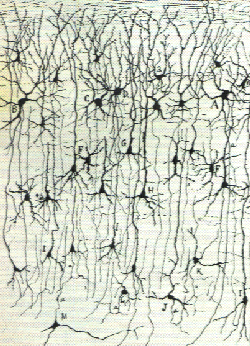3) Discretization of information
Finally we must more effectively represent the "discretization" of the passage of information in a diagram. When information passes through the synapses, temporarly a kind of dense network is created, formed by all the points of intersection between the neurons.

### D-2- Circular network

Faced with these difficulties, the new idea origin of the system to represent chryzodes lies in the use of a CIRCLE, a RING calibrated as a ClOCK and in which we represent paths, circuits between a set of elements in interaction, by lines.
 For example, to illustrate the possible interactions between a set of 9 elements, we use a circle on which we determine 9 equidistant points placed on the circumference. We say its module is  9.  We then connect each of the 9 points with all the other elements. The possible interactions between each element are thus represented by vectors connecting the corresponding points.   By granting a numerical value to each of these points, we make possible to carry out a bijection of the set of real numbers on the circumference.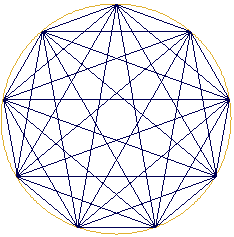ex. 1

This arithmetical special characteristic enables us to study the model starting from the laws governing the field of numbers. The way is tempting for, from quantum mechanics to the perception of harmonics of a violin, the arithmetical properties play a fundamental role.

D-3- Superposition of star polygons in various modules

Maintaining, on a same figure we superimpose modules having an increasing number of elements, that is to say integers series 2, 3, 4, etc.
 Thus, on the circle, to represent  number 2, we determine a point origin, then we place a second point which we connect by a line. To represent  number 3, 3 equidistant points are placed. One connects these 3 points and one obtains a triangle. Of course, it is useful to keep the same origin, just as the zero is used as starting point to study the scale of numbers. For number 4, one places 4 points which, when they are connected, draw a square more a cross, etc.  The figure thus obtained is represented opposite.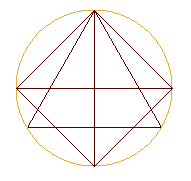ex.2
 Why not continue this process beyond 4 points? Curious as a researcher can be, we persevered and superimposed the set of the star polygons on our graph in a circumference divided into 4 points, 5 points, then 6, 7 points, like in the example 3 opposite.      ex 3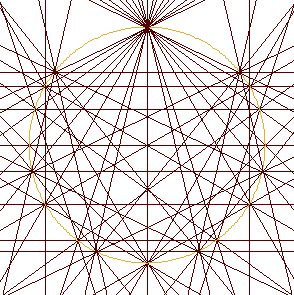Opposite, we then represented the integers until number 14. In other words, we carried out the superposition of all the star polygons in a circle divided into from 2 to 14 points.   Very quickly we here discover a new type of representation.  ex.4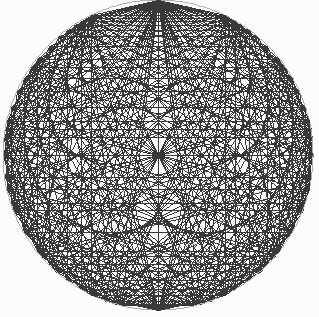In a more drastic way, we note that superimposing the networks highlights a series of hypocycloidic envelopes (within the circle). Extending the lines out of the circle, we should also have observed the presence of epicycloidic curves (external to the circle).  It should be noted that this type of structure does not seem to be fractal a priori (in the strict sense) for, if one extends the graph, one always discovers the fitting of straight and caustic lines, but does not get the  departing structure.  ex.5The instrument makes possible to carry out and  discover innumerable new figures. Also, the team of search with which I work on the subject, proposes that one calls these graphes chryzodes (1987). This word "CHRYZODE" is deduced from "chryzos" ( writing out of gold) and "zoi" (circle). It would mean a golden writing  on a circle. For us, it designates the large family of shapes resulting from the representation of numbers by means of vectors (and of intersections of these vectors) inscribed on a graduated circumference.

### D-4- First stage: SCAFFOLDING

Let us come back to the previous example to see that the extreme tangle of the network suggestes an underlying logical ordering. For that purpose, applying the arithmetical series to the system makes emerge as a kind of basic alphabet . Its handling enables to discover a very rich world hardly explored. It gives us  informations too about the structures, the resonances, the harmonies and the harmonics of the undulatory systems.

First let us here make out two fundamental stages at least : the construction and the points of intersection.
For the construction we place "m" equidistant points on the cicumference of a circle (m represents a number). We then connect these points by lines according to an arithmetical operation.

Multiplication by 2 in number 19
Example
Multiplication by 2 in number 19 : that is to say the geometric representation in a circle divided into m equal parts of a series of numbers defined by a multiplicative progression (in geometric progression).

We call that number m "module-circle m" or "circular referential in value m". We simply say it represents the module m. The example below illustrates m= 19.
To draw this series of doubles, we use a circumference on which we first have defined m equidistant points numbered from 1 to m.
Two representations can be used :
- either on the circle we connect each element to its double,
- or we connect the points of the circumference by lines segments according to the order of the terms of the series.
The progression of doubles theorically infinite will of course here be limited by the number m. Thus, each time the value of a term of the series is higher than the value of m, the value of m is substracted as many times as necessary from this term. (One does not differently count the hours when the 26 th hour is equivalent to 2 hours: on a clock in 24 elements we have counted 26 - 24 = 2 hours.). In mathematics that is said the terms of the series are congruent to the module m.
 In this way the progression of doubles 1, 2, 4, 8, 16, 32, 64, 128, etc., congruent to number 19, becomes a periodical series of 18 terms: 1, 2, 4, 8, 16, 13, 7, 14, 9, 18, 17, 15, 11, 3, 6, 12, 17, 10, 1, 2, 4, ...  On the circle we draw a line which goes from the point 1 to the point 2, another one from the point 2 to the point 4, and thus, until all the terms of the series are connected. Let's note the vectors are all tangent to a remarkable curve, the cardioide, and the network is hamiltonian, the point numbered 19 being also zero.                                    2*10=1 modulo 19 ex.6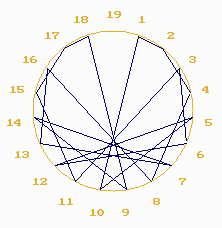Analysing the process let us note that from the 18th term the series has become periodical and that we are back at the departing point.
Going over this circuit in the opposite direction (1, 10, 5, 12, etc.) we notice that it corresponds to the series of multiples of 10 congruent to the module 19. In other words, we observe that, congruent to the module 19, the series of multiplications by 2 turns into the series of multiplications by 10.

In arithmetic, that is said 2 is the inverse of 10 in the module 19.
That is 2 * 10 = 1 modulo 19, this is here an expression of the Fermats' theorem
( when n is prime, n(m-1)=1 et nm-2=1/n ).

In a musical extrapolation, one would say that by number 19, the harmonies of 2 turn into harmonies of 10. Viewed from this angle, the chryzodes would seem to be like a representation, an image of this verse-inverse transformation or harmonization.
 Multiplication by 3 in number 19  Another example :  Let 's study the series of triples, that is : 1, 3, 9, 27, 81, etc.  Congruent to number 19, this progression of triples turns into a periodical sequence of 18 terms: 1, 3, 9, 8, 5, 15, 7, 2, 6, 18, 16, 10, 11, 14, 4, 12, 17, 13, 1, 3, etc.  Here, the inverses of the powers 3 are the powers 13  that is : 3 * 13 = 1 modulo 19. On the circle we draw a line which goes from the point 1 to the point 3, then another one from the point 3 to the point 9, and so on until all the terms of the series are connected. ex.7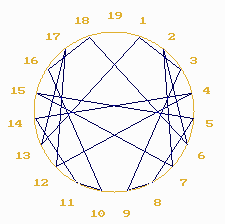Multiplication by 3 in number 211  Using a graduated circle with a larger number of elements, a better graphic definition of the present structure is obtained.   Opposite, is represented the series of triples in number 211.  3 * 142 = 1 MOD 211 ex.8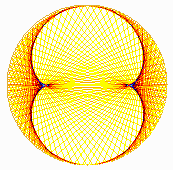Let us notice that with the multiplication by 3, the straight lines are all tangent to another cycloidal curve. This one, eight shaped, is called "nephroide".
Actually, every multiplicative progression in the chryzode generates a number of points of inflection with one unity less than the value of the progression. However, the case is different when the value of the series approaches the value of the number.
Thus, in the series of the multiples of 209 congruent to number 211, the shape will be epicycloidic, it will be a three external retrogressions astroide.

D-5- Second stage: POINTS OF INTERSECTION.

When long series of numbers are represented, the graph in lines becomes overloaded and it is no longer very exploitable. On the other hand, if one wants to illustrate the discontinuous, the corpuscular aspect of the system, it is shrewd to make only the points of intersection of the vectors of the chryzode in lines appear and that, by the means of computer.

 Multiplication by 3 in the number 2701    The example 9 opposite shows the points of intersection of the vectors resulting from the series of triples in number 2701. As regards the diffusion of the points on the graph, here one easily notices necks, moireings, etc shaped curves .  We soon make many discoveries and the chryzode in points of intersection takes an inedite relief both by its aesthetic aspect and by the properties it allows to show. ex9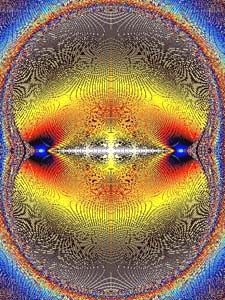D-6- Harmonic superposition of networks
Going on our investigations, we notice that the details of the shapes resulting from the multiplication by 3 depend on the value of the numbers in which the multiplication is represented.
Multiplication by 3 in the numbers multiple of 7  For example, opposite is the superposition of the chryzodes resulting from the multiplications by 3 in  some numbers multiple of 7 ( m = 7, 14, 21, 28, 35, etc.).  We thus observe the emergence of sorts of synchronization beams. The number of  beams is proportional to the pitch of the sequence (here 7). We could say that here  we go over the harmonies of 3 at the rate 7.  It is quite plausible to consider that the appearing beams relate to the harmonics of the vibratory system. (harmonics = multiple frequencies of a fundamental frequency 7, 14, 21, etc.) ex.10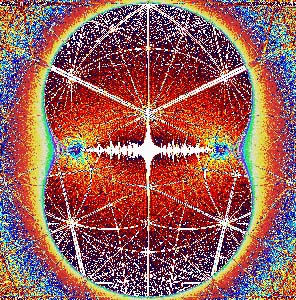Multiplication by 3 in number 7   Let us note the coincidences between the main beams of the chryzode in points in the example 10, and the lines of the chryzode in lines of the multiplication by 3 in number 7(opposite),that is the series 1, 3, 2, 6, 4, 5, 1. This synchronization is understable because the chryzode of the multiplication by 3 in number 7 is present in every superimposed elementary chryzode.  Viewed from this angle, the chryzodes in lines are comparable to a network, a generic graph, of the chryzode representing the harmonics networks.  3 * 5 =1 MOD. 7  ex 11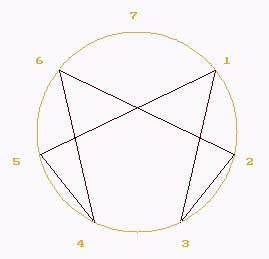Enlarging the multiplication by 3 in numbers multiple of 7  Enlarging a part from the example 10 we observe a sort of overlapping system linking the different beams between each other.  Example. 12 : a part of the ex. 10 enlargement ex.12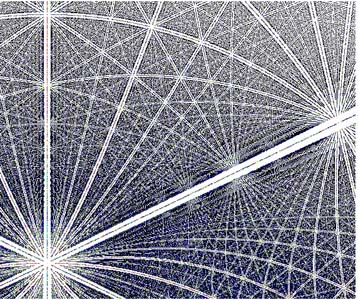D-7-1-Synchronization beams at a molecular level

As part of a cognitive approach, the dicovery and the modelization of the synchronization beams by superposition of networks are worth our attention. Actually, we have here like a bridge to better understand the properties of interconnections networks of the brain.  The graphic gain which the model offers is not to denigrate, for, synchronization beams almost identical to these of our model are found again in some other cases of networks superposition, such those obtained by x-ray cristallography of some proteins (Laue' Figures, see appendix D-a-1).  Opposite: Rubisco protein x-ray cristallography. ex13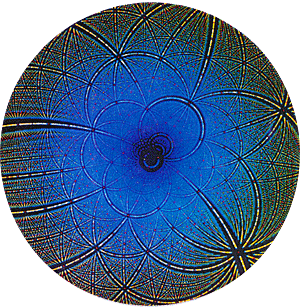D-7-2 Correlations between chryzode and Rubisco
 The possible correlations between two systems have encouraged us to try to find whether, by the means of the chryzodes system, we could get a figure approaching the one which is obtained in -ray-cristallography.   Opposite, one of the results. Let us note that we had to assign the rules of an homologic inversion to each point. ex14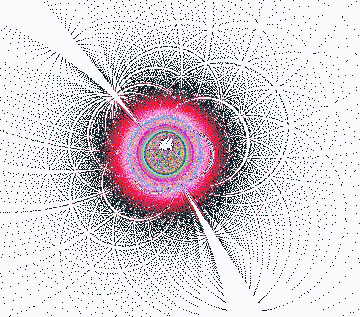D-8- The synchronization beam envisaged as a brick of a cognitive and behaviour management.
As a general rule, with the chryzodes system, the synchronization beams are found in every harmonic superposition of points networks.

The emergence of these beams in chryzodes and x-ray cristallography sets thinking. All the more so as our perception of visible has not get us used to such a type of structure while they appear quite quickly in the superposition of points networks (see appendix 3).Probably  if they are not directlly visible to the naked eye, it is that our visible perception covers only one frequency scale (from red to ultraviolet,there is only one multiplication by 2). Yet, the emergence of the beams is linked to an harmonic growth of the frequencies (ex: 2, 8, 16, etc.).
However, we know that the auditory cortex can analyse harmonics. The presence and the distribution of cells with a growing sensitivity in the internal auditory meatus prove that neurons are capable of a quantitative, qualitative and harmonic analysis. On the other hand, let's not forget that a part of the cortex used in the sound harmonics analysis can be in the deaf allied with some other cognitive phenomena.
Be that as it may, forced to deductive methods, it is interesting to envisage a sort of correlation between both phenomena, that is on one hand, the superposition of the cristallographic refractions, and on the other one, the modelization by means of the points of intersection of the vectors resulting from a superposition of networks.

At this stage, we have to consider that we have got a tool which gathers solutions to the three departing problems in an only scheme.

That is to say :
-The extreme density of the neuronal system : This one is resoluted by the fact that we are not limited in the quantity of the elements to take into account on the circumference. The analogy with the ring of  real numbers seems to be founded.
- The fitting of the treatment and the superposition: superimposing the various analysises on a same graph answers the difficulty. This enabled us to extract the synchronization beams.
-Discretization of information: using vectors as a scaffolding, and taking the only points of intersection into account, in a way just like at the intersection of a whole of neurons, we have entered a process partly similar to those we find in proteins x-ray cristallographies.

To extrapolate and to pursue towards the cognition mechanisms is a speculative venture which gets us ahead forward a few steps. However, and we quickly realize it, the task is colossal. The field of numbers is infinite, the shapes to be listed are also infinite. As for the classical mathematical tool, it proves to be incomplete to work out the whole of possibilities. We should have a super Fouriers' function, and the present approach towards the correlations by waves and the Gabors' function are in line with. As regards chryzodes, everything is still to be done, if we leave out the tracks left by Fermat, Pascal, Gauss and Poincare.
The bringing -in of the chryzodes makes new hypothesises likely and to be envisaged. For example during a cognitive analysis, the system sets up kinds of needles, axises or axises networks which take their origin in the synchronizations resulting from the superposition of the  various levels of the information processing. This can be as by an exciting effect of the axonal extremities as by an inhibiting effect. The beams may be isolated and fleeting like in the case of a small sense excitation or more intense, more concentrated, like in the case of a concentration or a philosophical meditation.

What matter to us is that these vectors, a little like paths, memory lines, "tubes" resulting from the synchronizations between the different layers operating an analyis, could be instantly or subsequently (REM sleep) used in a more vaste architectural set. We then should have a sort of "patterns" management, in the sense defined by Jung, molds, in a way a vocabulary and a grammar of cognition.

The difficulty is that in this type of investigation, it is quite difficult to go directly over to testing because of aspects linked to ethics. The more elevated in the scale of perceptions is the faculty we like to understand, the more it is necessary to go towards analysts, philosophers, visionaries in order to decipher their experiences in the light of our inquiring faith. Actually, the short-term experiences are not easily possible and the searches are long and complex. For example, before obtaining a chryzode looking like the Rubisco, we had to analyse the process  in a x-ray crystallography to note the fitting was generating an homological inversion.  On the other hand, a biologist advised us to present the images resulting from chryzodes to any light mentally deficients in order to study their reactions. Some mathematicians rose an outcry when we suggested this experimentation. It seemed to us useful then to avoid a conflictual approach.

Progressing in our research on chryzodes we have found multiple tracks. The correlation with the synchronization beams is only the one which seems to us the most reliable. Some other ways enable us to have a few chances to advance. In the appendix we present the ones seeming to us the most promising. A vast work remains to do. And though the chryzodes at this time seem to arise more questions than these they resolve, they at least have the advantage to offer us new senses for which had seem to be a labyrinth of complexity.

D-9- Epistemology

Remember, chryzodes make possible to explore every function operating on integer numbers and by approximation on real numbers.Whatever they are, recurring decimal or complicated equation decimals,they always show properties hidden by the abrupt austerity of an integro-differential formalism. This is a new way to representcertain mathematical objects, as lines and points networks, to show logical abstractions, to manipulate a sort of alphabet of shapes.

Considering the fertility of the obtained aesthetic and rationnal results, the system seems to be situated at an epistemologic croosroads and seems to be able to generate a whole of possible senses. They can nourish the everyone's imagination and creativity as in the field of research as in the field of experimentation as well in the growing one of modelling.
We first note that from a simple arthmetico-geometrical mechanism we get harmony, organization, but neither disorder, nor accident. The chryzodes make possible to approach arithmetic under a new angle of view, especially number in its simple operations, in the field of congruences or sequences.

Another important aspect seems to be the use of a circular referential that enables to envisage a geometry of chryzodes in complement of the analyticespecially to account for a process or a transformation. At the first glance, we understand the power of representation of the model : the economy in the contained information. The analogy with the ideal of the Greek mathematics in its research of alliance between proportion and beauty here is undeniable. We achieve the mathematical aspect of the model recognizing it as belonging to the new branch opened by, among others, Poincare, Julia, Thom or Moulin, and concerning chaos, disorder, catastrophes, fractals, arithmetic relators. This conception incontestably draws near a new epistemology with the consideration of reality as a possible "constructed" rather than a "given" reflecting an unchanging reality. The creation of patterns is one of the important instruments of this movement and the system of chryzodes seems to be able to favour simulations when it 's the matter of phenomena of a periodical or undalory nature.
With regards to the creation of shapes, one can think of an important bringing to morphogenesis,  understanding of processes and representation of waves fields.

Appendices
D-a-1- Epicycloides and hypocycloides

Definition :
"A point M on a mobile circle C with radius r (generator circle) which rolls without sliping on a fixed circle with radius (directrix) describes out a curve L. L is called epicycloide when the contact is external and hypocycloide when the contact is internal.
When the ratio R/r is a fraction which is in its irreducible form p/q (q different from 1), then the epicycloide is an algebraic curve too and it is composed of p equal branches." History
"The astronomers in ancient Greece explained the retrograde movements of planets giving them an  uniform movement following a circumference (epicycle) the center of which moves on another circumference. The property according to which the point of a circumference internally rolling on a motionless circumference with a radius two times larger describes the diameter of the motionless circumference, was independently discovered by Nassir Eddin (middle 13th-century) and Nicolas Copernic (beginning 16th).
But the systematic study of the epicycloide and  hypocycloide started in 1525 with the Albrecht Dürer's theoretical works that remained unknown from mathematicians.
Desargues and then La Hire, at the middle of the 17th-century, worked out many important properties of these curves.
In its "Mathematical principles in the natural philosophy" (1687), Newton generalized the Huyghens's researches about the cycloïdal pendulum and he worked out that in a spherical attraction field, the isochronal oscillations of the pendulum is the epicycloïde.
Afterwards the natural generalization of cycloïdes, epicycloïdes and hypocycloïdes did not stop attracting the searchers'attention, among them, Leibnitz, Euler et Bernouilli."

Texts from "Aide mémoire de mathématiques supérieures", M Vygodsky, ed. de Moscou, 1973.

D-a-2-Method of diffraction in polychromatic radiation

### There is only  one method of diffraction in polychromatic radiation and it only applies to monocrystals: this is the Laue method. A tungsten anticathode A excited under a  tension of 50 to 80 kilovolts is used. We thus have a continuous spectrum included between between 0,2 and 2 A. An approximately parallel beam is obtained by means of two holes a few tenths millimeters wide pierced in two lead plaques assembled at the extremities with a metal tube. The beam falls on the crystal and the Laue patches form on the sensible surface S. The  distances from A to D1 and from D1 to D2 approximately are of 4 or 5 cm. The crystal C is very near from the  diaphragm D2. The distance CO, from C to S varies between 2 and 10 cm. Each patch characterizes a family of reticular planes, except the central patch generated by the direct beam which came across the crystal without diffracting it. If in the spectrum produced by the anticathode  there is a wavelength that verifies the Braggs 'hypothesis, there is a beam selectively reflected under the angle COT (T: Laue patch). (...)

The Laue method does not give any informations about the reticular distances but only about the angles.

In order to show the polychromatic nature of Laue diagrams, we carried out some colour ones with the following artifice.
Three diagrams of a same crystal are taken at different tensions: one at 18 kilovolts without filter, the second one at 35 kilovolts with an aluminium filter of 2 mm, the third one with an aluminium one of 4 mm. We thus select three ranges of wavelengths that would respectively correspond to the orangy red, green-yellow and purple-blue regions of the visible spectrum, if they could be multiplied by 10 000. We artificially operated this transposition using the trichromatic synthesis: each one of these snaps is printed in a suitable colour and their superposition gives a diagram the patches of which go from red to purple when the radiation which produced them goes from 18 to 55 kilovolts.

Texts taken from: " Principes de radio-cristallographie" by Jean Barraud, Masson ed., 1960, p 84 to 87

D-a-3- Details of a superposition of networks.

Next we study two asymptomatic scenarios , that is:

1) Superposition of networks in modules multiple of a same number.
2) Superposition of networks in modules of consecutive values.

1) Superposition of networks in modules multiple of a same number.
In the example below each layer illustrates an increasing number of elements in interaction.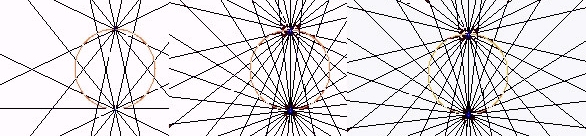Then, let us substitute for each network in lines its network in points of interconnection.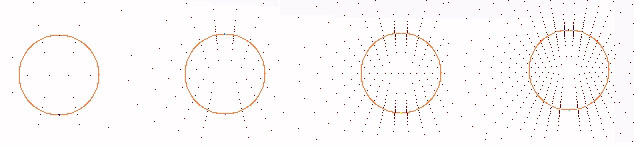Although each layer does not separately seem to have a particular structure, on a third graph we observe that the superposition of five points networks already makes a small set of synchronization beams emerge.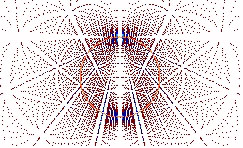Carrying on the process a few steps further, this one gets accentuated.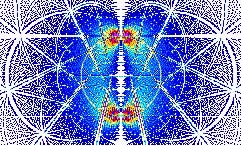2) Superposition of networks in modules of consecutive values
With a small interval (or not) between each number, the harmonic beams disappear giving the place to kinematics of whirling shapes made of a tang of waterings, curves, necks, nodes, focuses.

Multiplication by 3 in the numbers 1000, 1001, etc.
If we give a different colour to each number and we make the palette of colours turn, we easily observe the movement of the diverse whirlwinds. The waterings (necks, curves in layers, etc.) seem to correspond to the partials of the wave (partials = groups of frequencies, the values of which are consecutive ex 1001, 1002, 1003, etc.). Ex below: the details of the superposition of the multiplication by 3 in circles with from 1000 to 1200 elements.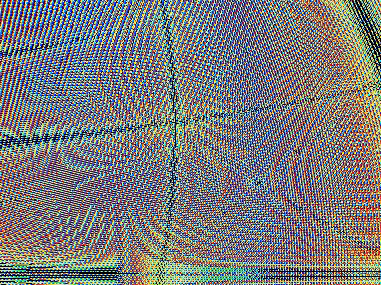Of course some other powers than these of 2 or 3 can be expressed. To vary the type of operation and the type of function leads to discover an infinity of figures. This infinity of possible topologies and the cybernetic levels that we easily detect would themselves require a thesis. In fact, many other aspects are yet to be resolved. Let us sum up saying the chryzodes graphically express arithmetical properties that can be extended to the reals numbers by approximation. As for the structures emerging from the different superpositions of networks , they are found in physics in many resonance phenomena , like in the structures resulting from x-ray cristallography or, we give an example below, in the luminous refractions produced by a vibrating circular mirror.
D-a-4 Vibrating circular mirror
A simple example allows to approach the physical phenomena generating figures linked to the shapes discovered by chryzodes.

Let us stand a wide circular container with a little liquid in on an unstable rest . For example a big aluminium pan with a little water inside and put on a rickety table. We light this container with a luminous beam in such a way that the luminous refractions coming from the pan are reflected on the ceiling of a dark room. At rest, we observe a simple shimmering as it happens at the surface of an expanse of water.  Let's now animate the table with the pan by a rythmic motion.   We then observe a sort of rose with an hypocycloidic contour, on the ceiling of the room, in the drawing of the reflections coming from the container set on the table.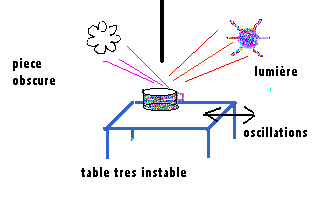Let's change the frequency of our motion, the luminous phenomenon turns into a spiral, then it is transformed again into a new rose with a different number of petals, once the motion is stabilized on another frequency.

D-a-5-Superposition of couples of inverses
Vertically and horizontally going over the table of the multiples congruent to a number, chryzodes relate kinematics of epi and hypocycloidic topologies. Each one of these shapes is itself made of all a fractional cycloides spectrum. These different oscillations between the intern and the extern according to some processes of implosion-expansion can the most of the time be summed up by the couple of inverses : a * b = 1 mod m.

At a superior level of representation, instead of drawing all the transformation signified by a chryzode resulting from a series of powers , we can identify the process by a simple line connecting the verse term to its inverse, on a new chryzode.
In the example 6 previously seen and that relates the power of 2 transformations into powers of 10 when they are congruent to number 19, we only have to connect the point 2 to the point 10 on a circumference divided into 19 points.
It is then useful to represent the other verse-inverse couples of number 19, what is easily obtained by calculating the powers N-2 of the number.
 For 1 2 3 4 5 6 7 8 9 10 11 12 13 14 15 16 17 18, etc., we have:   1 10 13 5 4 16 11 12 17 2 7 8 3 15 14 8  that are the verse-inverse couples:   2*10, 3*13, (4*5), (5*4), (6*16), etc.   In a new chryzode, these different couples are represented by the means of vectors connecting the terms of each couple.  A more exhaustive study makes the chryzode appear modelizing the couples of inverses as a sort of "identity card" summing up a certain number of arithmetical properties of  number 19.Contrary to the chryzodes resulting from the multiplications in which the presence of hypocycloidic curves seem to be an invariant, the structures of the chryzodes representing the couples of inverses seem to be geometrically different from each other.
Below, we have represented the couples of the inverses of numbers 232 to 235.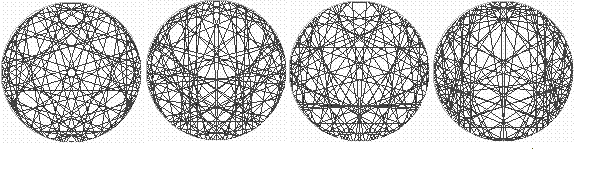Yet, and surprisingly, constructing the superposition of the couples of inverses of a quite large sequence of consecutive modules, we notice the multitude of distinct shapes is joined, jointed in an unique structure.  Opposite, see the superposition of the identity chryzodes from 5 to 50 in a same circle.Finally let us say that this type of new shape looks a little like a shell in the first stages of its construction, but very quickely this shape fades for a topology not yet classified.  Example opposite: we have superimposed every vector representing the couples of inverses of the numbers from 5 to 236.In an attempt of an exhaustive approach of the field, this topology would seem to be like a fundamental lighthouse, a sort of informative field skeleton, a sort of central pivot. The poet would maybe see here a key giving the access to many other topologies, that could be to some extent called computing, so much close are the interrelations to the image of the interrelations between the different levels of the mathematical abstraction.

D-a-6 Comparison between the Helmotz curve and chryzode

Finally let's cite an interesting parallel between the position and the intensity of the internal peaks of inflection of the star polygons superposition in a chryzode (split into two) and the Helmotz consonance curve. The latter is worked out from the harmonious concordance between a C sound and another sound generated by a musical string going from this C up to the C of the superior octave.
 We note the resonance troughs of the Helmotz 's graph correspond to the half-chryzode peaks of inflection. Thus, we discover a surprising correlation of structure between the peaks of inflection of the hypocycloidic curves of the chryzode representing the integers series and the resonance chords of the musical scale (represented by the troughs of the helmotz's curve) in relation to the initial C.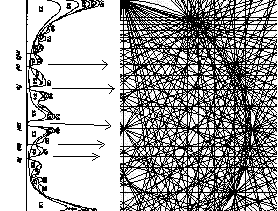### Concluding the appendix D

The chryzodes described in this presentation only are the first letters of an alphabet of shapes and many other structural processings are applicable for each aspect of a structural resonance. In life experience, the organization of things is much more subtle than all the possible rationalizations (fortunately!). Life modifies models, moulds, at its convenience, however respecting some constraints. Yet, for as simple as the chryzodes are, they are not to be neglected: they have many analogies of organization in relation to our logic of perception and in the present neurophysiological research diverse indications are found tending to support the incidence of such an organization within our brain. The similitude of the vectorial decodings must not escape us, all the more so as chryzodes are themselves a logical way of decoding. Apparently, the visual cortex of monkeys shows a marked preference of analysis for the angles 10 degrees apart. In a chryzoidic application, we could say the monkey's prefered relator is a chryzoidal ring with 32 different angles, that is an exponential base (power of..) 37. This is only a rudimentary way of envisaging some more succinct fields of application.

BIBLIOGRAPHY OF THE APPENDIX D
"Le Cerveau" ; Ed. Pour la Science, nov. 1979.
"La Recherche", Laure Schachli, december 1991.
" Principes de radio-cristallographie", Jean Barraud, Masson ed., 1960, p 84 to 87.
"Les Nombres Premiers " ; J. Itard : PUF.
"Hypocycloïdes et Epicycloïdes" ;J. Lemaire : Libr. Blanchard.
"Théorie des Nombres" ; E. Lucas : Librairie Albert Blanchard.
"Aide Mémoirede Mathématiques Sup." ; M. Vygodski : Ed. Mir.
"Aide Mémoire de Physique" ; B. Yavorski et A. Detlaf :Ed. Mir. "Actes Congrès MATh. en. JEANS. 1996", Pierre Duchet ; ed MATh en JEANS, Paris
"Eurêka",Helène Prost, décembre 1995, Bayard Presse, Paris
"Encyclopedia of World Problems & Human Potential ", Anthony Judge, 1994, ed U.I.A., Bruxelles
"Journées nationales APMEP 1993", Pierre Germain, Jean-Paul Sonntag, ed. APMEP, Paris
"Science en Fête 1993", Corinne Rouiller, Pierre Germain, Jean-Paul Sonntag, Ministère de la Recherche, Paris
"Second European Congress on Systems Science in Prague- Proceedings", 1993, ed. UES.-AFCET, Pierre Germain, Corinne Rouiller.
"U.A.T.I., n 1", Marcel Locquin, 1993, ed. UNESCO,
Actes Congrès "MATh. en. JEANS. 1993", Pierre Audin, ed MATh.en.JEANS, Paris
"Actes Congrès International UITF 1992", Corinne Rouiller, Jean-Paul Sonntag, ed UITF, Paris
"Actes Colloque Systema 1992", Pierre Germain, Jean-Paul Sonntag, AFCET, Paris
****